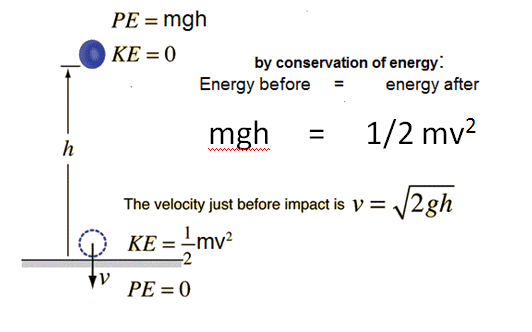## The Conservation of Energy

The conservation energy is a fundamental concept in physics. "Energy can neither be created nor destroyed", just transformed from one type to another. The application of the conservation of energy provides a powerful tool for problem solving.

The conservation of energy helps us determine how much kinetic energy an object has.The energy has all come from the gravitational potential it has before falling,so it is :

E=mgh

Where :  (m : the mass of the object) / (E is the energy) /(g is the acceleration due to gravity constant 9.81 m s−2) / ( his the height)The Work-Energy Principle

The work-energy principle is the last needed thing to work al this out. This principle states that:

Average impact force × Distance traveled = Change in kinetic energy

Since we are looking for the average impact of force , this equation can be rearranged as so :

Average impact force = Change in kinetic energy ÷ Distance traveled

And since the change in kinetic energy is the same as the gravitational potential energy, the complete formula can be expressed as:

Average impact force = mgh ÷ d

Calculating example

Supposing we want to know the impact force of a rock with a mass of 1 kilogram that falls from a height of 2 meters and embed itself 4 centimeters deepinside of a plastic toy.  Remembering that the average impact force = mgh ÷ d, we put the example figures in place, The force of impact from the rock will be:

Average impact force = (1 kg × 9.8 m s−2 × 2 m) ÷ 0.04 m = 490 Newtons.